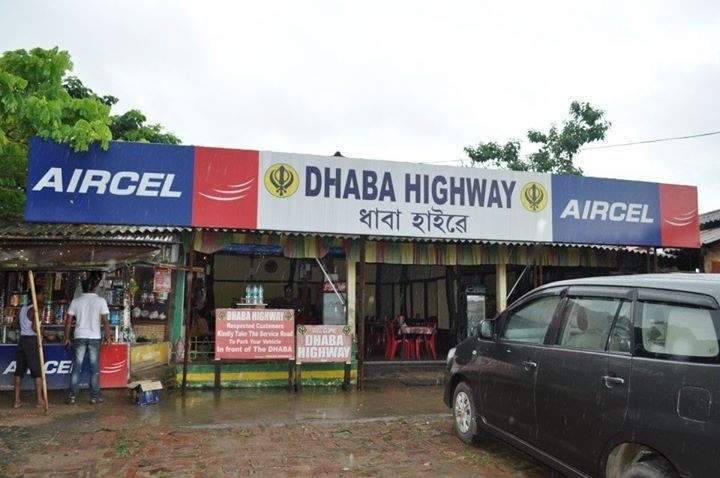How Cheenta works to ensure student success?
Explore the Back-Story

# The Dhaba Problem | ISI and CMI EntranceSuppose on a highway, there is a Dhaba. Name it by Dhaba A.

You are also planning to set up a new Dhaba. Where will you set up your Dhaba?

Model this as a Mathematical Problem. This is an interesting and creative part of the BusinessoMath-man in you.

You have to assume something for Mathematical simplicity to model a real life phenomenon via math.

Assumptions:

• The length of the highway is 1 unit. Assume the highway is denoted by the interval [0,1].
• The number of Persons in a road is proportional to the road length. P = c. R, where P is the Persons and R is the road length, c is an arbitrary constant.
• The profit is modeled as the Total Number of Persons arriving in the Dhaba.
• A person will visit a dhaba which is nearer over another which is further away.
• When a customer is at the same distance from two shops then the customer choose randomly.

Observe that the assumptions are valid and are actually followed in real life. Just sit and think for some time placing yourself in that position.

The Explicit Mathematical Problem

Profit Calculation of your Dhaba B

Now based on the lengths of the roads above and the assumptions made, we have to calculate the profit of Dhaba B.

So the profit made by B = c.R , where R is the length of the road on which B has monopoly. Here, as shown R = \c.( x + \frac{|d-x|}{2}\).

We have assumed in this case that $0 \leq x \leq d$ by the diagram.

So, the profit for the Dhaba B is c.$\frac{d+x}{2}$ .

Hence as $0 \leq x \leq d$, the profit is maximized if x = d.

Exercise: Show that the profit of Dhaba B as a function of x is c.($1 - \frac{d+x}{2}$) if $d \leq x \leq 1$ .

Also, observe that in this case the profit is maximized at x = d.

Exercise: Calculate the maximum profit in both cases. Do you observe something fishy? What steps and what arguments will you give to understand the fishiness?

Eager to listen to your beautiful ideas.

Suppose on a highway, there is a Dhaba. Name it by Dhaba A.

You are also planning to set up a new Dhaba. Where will you set up your Dhaba?

Model this as a Mathematical Problem. This is an interesting and creative part of the BusinessoMath-man in you.

You have to assume something for Mathematical simplicity to model a real life phenomenon via math.

Assumptions:

• The length of the highway is 1 unit. Assume the highway is denoted by the interval [0,1].
• The number of Persons in a road is proportional to the road length. P = c. R, where P is the Persons and R is the road length, c is an arbitrary constant.
• The profit is modeled as the Total Number of Persons arriving in the Dhaba.
• A person will visit a dhaba which is nearer over another which is further away.
• When a customer is at the same distance from two shops then the customer choose randomly.

Observe that the assumptions are valid and are actually followed in real life. Just sit and think for some time placing yourself in that position.

The Explicit Mathematical Problem

Profit Calculation of your Dhaba B

Now based on the lengths of the roads above and the assumptions made, we have to calculate the profit of Dhaba B.

So the profit made by B = c.R , where R is the length of the road on which B has monopoly. Here, as shown R = \c.( x + \frac{|d-x|}{2}\).

We have assumed in this case that $0 \leq x \leq d$ by the diagram.

So, the profit for the Dhaba B is c.$\frac{d+x}{2}$ .

Hence as $0 \leq x \leq d$, the profit is maximized if x = d.

Exercise: Show that the profit of Dhaba B as a function of x is c.($1 - \frac{d+x}{2}$) if $d \leq x \leq 1$ .

Also, observe that in this case the profit is maximized at x = d.

Exercise: Calculate the maximum profit in both cases. Do you observe something fishy? What steps and what arguments will you give to understand the fishiness?

Eager to listen to your beautiful ideas.

This site uses Akismet to reduce spam. Learn how your comment data is processed.

### Knowledge Partner